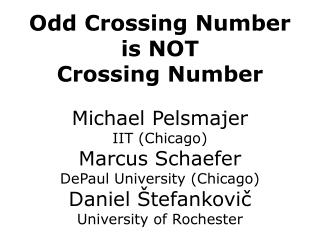DownloadDownload PresentationOdd Crossing Number is NOT Crossing Number

# Odd Crossing Number is NOT Crossing Number

Télécharger la présentation## Odd Crossing Number is NOT Crossing Number

- - - - - - - - - - - - - - - - - - - - - - - - - - - E N D - - - - - - - - - - - - - - - - - - - - - - - - - - -
##### Presentation Transcript

1. Odd Crossing Number is NOT Crossing Number Michael Pelsmajer IIT (Chicago) Marcus Schaefer DePaul University (Chicago) Daniel Štefankovič University of Rochester

2. Crossing number cr(G) = minimum number of crossings in a planar drawing of G cr(K5)=?

3. Crossing number cr(G) = minimum number of crossings in a planar drawing of G cr(K5)=1

4. Rectilinear crossing number rcr(G) = minimum number of crossings in a planarstraight-line drawing of G rcr(K5)=?

5. Rectilinear crossing number rcr(G) = minimum number of crossings in a planarstraight-line drawing of G rcr(K5)=1

6. Rectilinear crossing number rcr(G) = minimum number of crossings in a planarstraight-line drawing of G cr(G)  rcr(G)

7. cr(G)=0  rcr(G)=0 THEOREM [SR34,W36,F48,S51]: Every planar graph has a straight-line planar drawing. Steinitz, Rademacher 1934 Wagner 1936 Fary 1948 Stein 1951

8. Are they equal? cr(G)=0 , rcr(G)=0 cr(G)=1 , rcr(G)=1 cr(G)=2 , rcr(G)=2 cr(G)=3 , rcr(G)=3 ? cr(G)=rcr(G)

9. cr(G)  rcr(G) THEOREM [Guy’ 69]: cr(K8) =18 rcr(K8)=19 cr(G)=rcr(G)

10. cr(G)  rcr(G) THEOREM [Guy’ 69]: cr(K8) =18 rcr(K8)=19 THEOREM [Bienstock,Dean ‘93]: (8k)(9G) cr(G) =4 rcr(G)=k

11. Crossing numbers cr(G) = minimum number of crossings in a planar drawing of G rcr(G) = minimum number of crossings in a planarstraight-line drawing of G cr(G)  rcr(G) (G) cr(G)  rcr(G)

12. Odd crossing number ocr(G) = minimum number of pairs of edges crossing odd number of times

13. Odd crossing number ocr(G) = minimum number of pairs of edges crossing odd number of times ocr(G)  cr(G)

14. Odd crossing number ocr(G) = minimum number of pairs of edges crossing odd number of times ocr(K5)=?

15. Proof (Tutte’70): ocr(K5)=1 INVARIANT: How many pairs of non-adjacent edges intersect (mod 2) ?

16. Proof (Tutte’70): ocr(K5)=1

17. Proof: ocr(K5)=1 How many pairs of non-adjacent idges intersect (mod 2) ? steps which change isotopy:

18. Proof: ocr(K5)=1 How many pairs of non-adjacent idges intersect (mod 2) ? steps which change isotopy:

19. Proof: ocr(K5)=1 How many pairs of non-adjacent idges intersect (mod 2) ?

20. Proof: ocr(K5)=1 How many pairs of non-adjacent idges intersect (mod 2) ? QED

21. Hanani’34,Tutte’70: ocr(G)=0  cr(G)=0 If G has drawing in which all pairs of edges cross even # times  graph is planar!

22. Are they equal? ocr(G)=0 , cr(G)=0 QUESTION [Pach-Tóth’00]: ? ocr(G)=cr(G)

23. Are they equal? ocr(G)=0  cr(G)=0 ? ocr(G)=cr(G) Pach-Tóth’00: cr(G)  2ocr(G)2

24. Main result THEOREM [Pelsmajer,Schaefer,Š ’05] ocr(G)  cr(G)

25. How to prove it? THEOREM [Pelsmajer,Schaefer,Š ’05] ocr(G)  cr(G) • Find G. • Draw G to witness small ocr(G). • Prove cr(G)>ocr(G).

26. How to prove it? THEOREM [Pelsmajer,Schaefer,Š ’05] ocr(G)  cr(G) • Find G. • Draw G to witness small ocr(G). • Prove cr(G)>ocr(G). Obstacle: cr(G)>x is co-NP-hard!

27. Crossing numbers for “maps”

28. Crossing numbers for “maps”

29. Crossing numbers for “maps”

30. Ways to connect

31. Ways to connect

32. Ways to connect

33. Ways to connect

34. Ways to connect

35. Ways to connect number of “Dehn twists” -1 0 +1

36. Ways to connect How to compute # intersections ?

37. Ways to connect How to compute # intersections ? 0 2 1

38. Crossing number min i<j|xi-xj+(i>j)| xi2Z do arcs i,j intersect in the initial drawing? the number of twists of arc i

39. Crossing number i min i<j|xi-xj+(i>j)| xi2Z j do arcs i,j intersect in the initial drawing? the number of twists of arc i

40. Crossing number min i<j|xi-xj+(i>j)| xi2Z j i do arcs i,j intersect in the initial drawing? the number of twists of arc i

41. Crossing number min i<j|xi-xj+(i>j)| OPT xi2Z OPT* xi2R

42. Crossing number min i<j|xi-xj+(i>j)| OPT xi2Z OPT* xi2R Lemma: OPT* = OPT.

43. Crossing number min i<j|xi-xj+(i>j)| Lemma: OPT* = OPT. Obstacle: cr(G)>x is co-NP-hard!

44. Crossing number min i<j|xi-xj+(i>j)| yij¸ xi-xj+(i>j) yij¸ –xi+xj-(i>j) Obstacle: cr(G)>x is co-NP-hard!

45. Crossing number min i<j yij yij¸ xi-xj+(i>j) yij¸ –xi+xj-(i>j) Obstacle: cr(G)>x is co-NP-hard!

46. Crossing number Dual linear program max i<j Qij(i>j) QT=-Q Q1=0 -1  Qij  1 Q is an n£n matrix

47. EXAMPLE: a b c d

48. Odd crossing number ? a b c d

49. Odd crossing number a ocr  ad+bc b c d

50. Crossing number ? a max i<j Qij(i>j) b QT=-Q Q1=0 -1  Qij  1 c d =(2,1,4,3) a  b  c  d a+c  d 0 ac b(d-a) * -ac 0 ab a(c-b) b(a-d) -ab 0 bd * a(b-c) -bd 0 cr  ac+bd